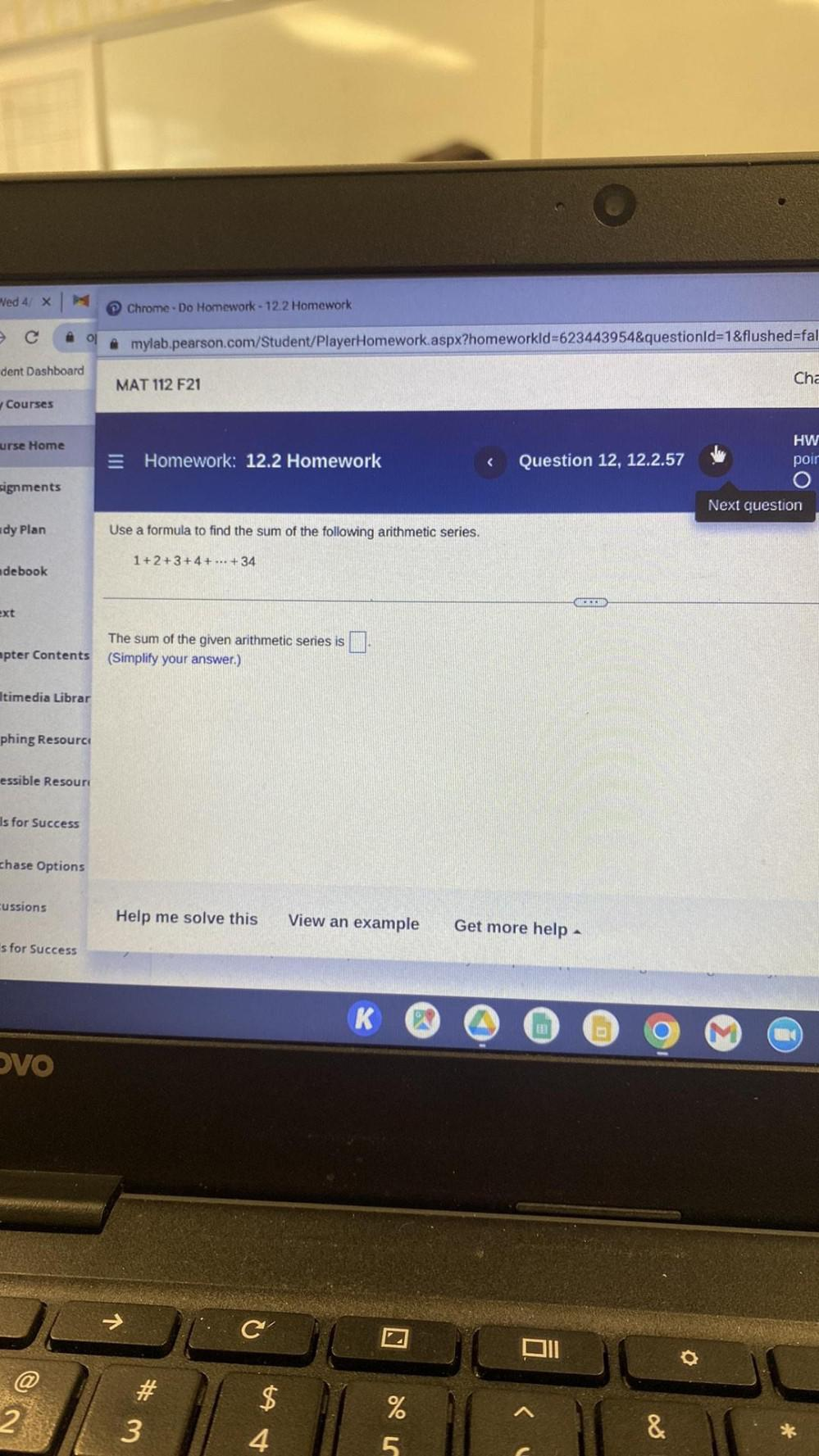Question:

# Use a formula to find the sum of the following arithmetic series. 1+2+3+4+ ... +34 The sum of the given arithmetic series is ▢ (Simplify your answer)Use a formula to find the sum of the following arithmetic series. 1+2+3+4+ ... +34 The sum of the given arithmetic series is ▢ (Simplify your answer)